Next: C.1 The Infinite Potential Up: Dissertation Christoph Wasshuber Previous: B Fermi Energy Dependence

# C Solutions to Schrödinger's Equation

The time-dependent Schrödinger wave equation which describes the motion of a particle under the influence of a potential, reads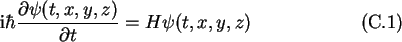We are interested in the motion of a particle in a potential landscape. Thus the Hamilton operator is replaced with the Hamilton operator for the free electron plus the potential function describing the potential landscape.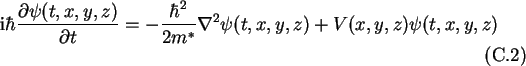It should be noted that the time-dependent Schrödinger wave equation is, in fact, a diffusion' not a wave' equation, the time derivative, being of the first and not of the second order. A wave equation and a diffusion equation have the form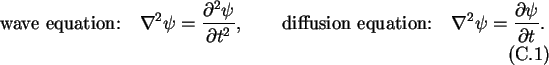Thus indeed the Schrödinger equation (C.2) has the form of a diffusion equation. With the separation Ansatz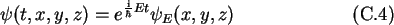one derives the time-invariant one-dimensional Schrödinger equation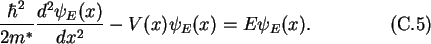For an easier notation in the following examples,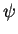stands for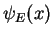.

Christoph Wasshuber